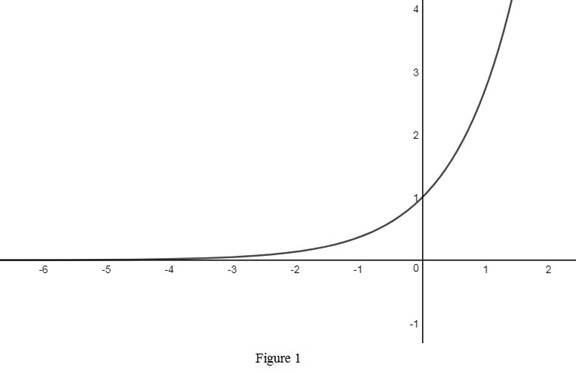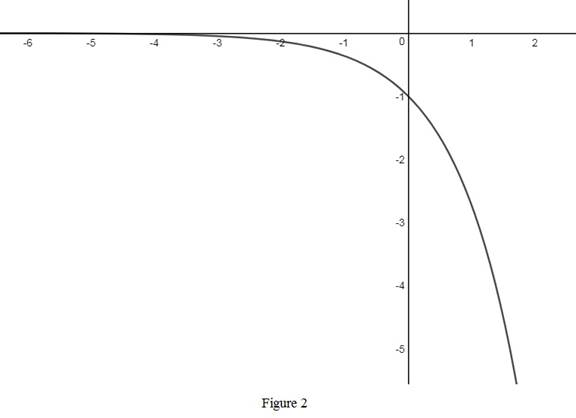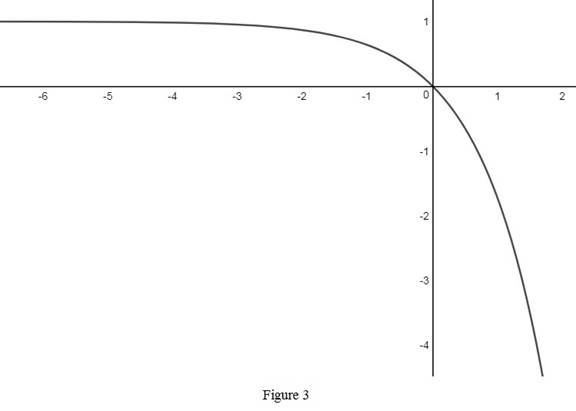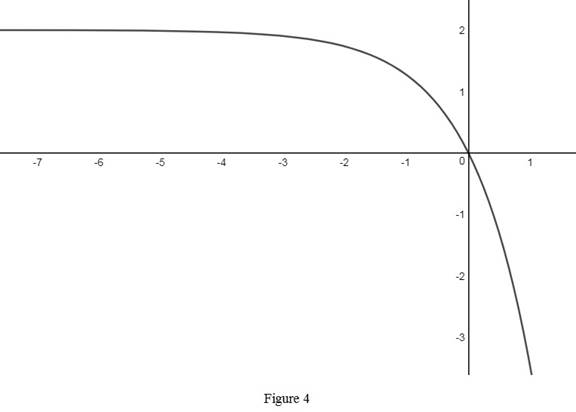# The rough graph of the function y = 2 ( 1 − e x ) use transformations if needed.### Single Variable Calculus: Concepts...

4th Edition
James Stewart
Publisher: Cengage Learning
ISBN: 9781337687805### Single Variable Calculus: Concepts...

4th Edition
James Stewart
Publisher: Cengage Learning
ISBN: 9781337687805

#### Solutions

Chapter 1.5, Problem 16E
To determine

## To sketch: The rough graph of the function y=2(1−ex) use transformations if needed.

Expert Solution

### Explanation of Solution

The standard graph of the function y=ex is roughly sketched as shown below in Figure 1.Reflect the graph y=ex about the x-axis and obtain the graph of y=ex . Thus, the graph of y=ex is drawn and shown below in Figure 2.Then, to draw the graph of y=1ex , shift the graph y=ex one unit upward. Thus, the graph of y=1ex is shown below in Figure 3.Then, draw the graph of y=2(1ex) , by stretch the graph y=1ex vertically by a factor of 2. Thus, the graph of y=2(1ex) is shown below in Figure 4.Thus, the required graph y=2(1ex) is obtained from the basic graph y=ex by performing some transformations.

### Have a homework question?

Subscribe to bartleby learn! Ask subject matter experts 30 homework questions each month. Plus, you’ll have access to millions of step-by-step textbook answers!